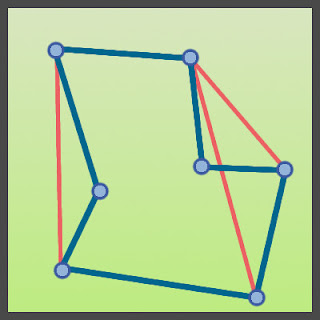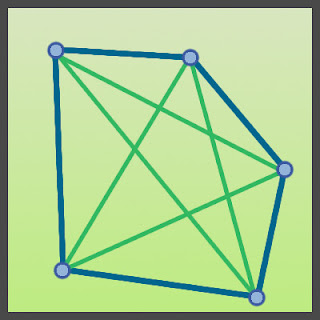## Convex Hull Algorithm

Convex Hull algorithms are one of those algorithms that keep popping up from time to time in seemingly unrelated fields from big data to image processing to collision detection in physics engines, It seems to be all over the place. Why should you care? Cus you can do magic with it and it seems so simple to implement when you first hear about it, but when you start thinking about it, you will realize why it's not such a straightforward thing to do.
Now that I got you interested (hopefully) and now we will see just what a convex hull is.
As you may have noticed a perimeter was made with the same points that was given and these perimeter points enclose the entire set of points.
Now we have to clear up the term 'Convex'.
Convex means no part of the object is caved inwards or that none of the internal angles made by the points exceed 180 degrees.An example of concave shape
In this example of a concave shape internal angles go beyond 180 degrees.
What are those red lines for? Well... concave shapes have a feature that can easily differentiate it from a convex one, in  the case of a  concave shape at least one line from one point to another will travel outside the shape or a line made by any two points will intersect with another set of lines making up the shape.
Those conditions don't apply to a convex shape.An example of convex shape
All the points can connect with any other point without intersecting any other line in the shape.
The 2D implementation of the Gift Wrapping algorithm is called 'Jarvis March'.
The image above describes how the algorithm goes about creating the convex hull. We will look at some pseudo code( based on the one given in Wikipedia )
``````CreateConvexHull(SetOfPoints)
pointOnHull = leftmost point in SetOfPoints
i = 0
repeat
P[i] = pointOnHull
endpoint = SetOfPoints // initial endpoint for a candidate edge on the hull
for j from 1 to |SetOfPoints|
if (endpoint == pointOnHull) or (SetOfPoints[j] is on left of line from P[i] to endpoint)
endpoint = SetOfPoints[j]  // found greater left turn, update endpoint
i = i+1
pointOnHull = endpoint
until endpoint == P   // wrapped around to first hull point
``````
You may have noticed that there are sections in the pseudo code regarding finding or checking if a point lies on the left.We will take a look at those instances.
``pointOnHull = leftmost point in SetOfPoints``
This line says that we should find the left most point, so if we are using the X-Y co-ordinate plane the left most point will be the point with the smallest X co-ordinate.
``if (endpoint == pointOnHull) or (SetOfPoints[j] is on left of line from P[i] to endpoint)  ``
We are checking if the point 'SetOfPoints[j]' is on the left of the line from 'P[i]' to 'endpoint'.
How do we checking if a point is on the left or right side of a line?
Here's how:
``(Bx - Ax) * (Cy - Ay) - (By - Ay) * (Cx - Ax) ``
'A' and 'B' are the points making up the line and C is the point we are checking whether it's to the right or left or if it's a point lying along line (collinear).
If we get the result as 0, then the point is collinear.
If we get the result as +ve, then the point is to the left
If we get the result as -ve, then the point is to the right.
This is end result we will be getting.
We are going to be making this so that I can be run in the editor, so we are going to be making use of Gizmos (debugging tool in unity) to draw the spheres and lines.
So now create a class called JarvisMarchConvexHull( very creative name 👏).
These are the member variables.
``````public Transform[] points;
[Range(0.05f,1.5f)]
public float size;
public bool drawIt;
private HashSet<Transform> result;
``````
'points' - the transforms that are going to be passed in from the inspector.
'size' - the size if the sphere to be drawn
'drawIt' - whether or not I want to display the spheres and lines.
'result' - this is a HashSet which means only unique items can be added to the list, It's useful when we have to avoid duplication or when we are trying to sound smart about data structures 😛.
*Warning Big Wall Of Text Incoming, Don't worry, we are breaking everything down into simple chunks below.
Let's looks at the Update function.
``````void Update()
{
result = new HashSet<Transform>();
int leftMostIndex = 0;
for (int i = 1; i < points.Length; i++)
{
if (points[leftMostIndex].position.x > points[i].position.x)
leftMostIndex = i;
}
List<Transform> collinearPoints = new List<Transform>();
Transform current = points[leftMostIndex];
while (true)
{
Transform nextTarget = points;
for (int i = 1; i < points.Length; i++)
{
if (points[i] == current)
continue;
float x1, x2, y1, y2;
x1 = current.position.x - nextTarget.position.x;
x2 = current.position.x - points[i].position.x;
y1 = current.position.y - nextTarget.position.y;
y2 = current.position.y - points[i].position.y;
float val = (y2 * x1) - (y1 * x2);
if (val > 0)
{
nextTarget = points[i];
collinearPoints = new List<Transform>();
}
else if (val == 0)
{
if (Vector2.Distance(current.position, nextTarget.position) < Vector2.Distance(current.position, points[i].position))
{
nextTarget = points[i];
}
else
}
}
foreach (Transform t in collinearPoints)
if (nextTarget == points[leftMostIndex])
break;
current = nextTarget;
}
}``````
We will go through all the important sections one by one.
``````
int leftMostIndex = 0;
for (int i = 1; i < points.Length; i++)
{
if (points[leftMostIndex].position.x > points[i].position.x)
leftMostIndex = i;
}
Here we are finding the left most point and adding it to the result which is the HashSet that holds all the points of the convex hull.
``````List<Transform> collinearPoints = new List<Transform>();
Transform current = points[leftMostIndex];``````
We need list of points that are collinear to the line we are checking currently and then do some stuff with them - remember this, we will come to this later.
And we are creating a new variable called 'current' which will be used in the loop to signify which point we are on right now. We assign current to the left most point in the beginning.
Now the loop.
``````while (true)
{
Transform nextTarget = points;
for (int i = 1; i < points.Length; i++)
{
if (points[i] == current)
continue;
float x1, x2, y1, y2;
x1 = current.position.x - nextTarget.position.x;
x2 = current.position.x - points[i].position.x;
y1 = current.position.y - nextTarget.position.y;
y2 = current.position.y - points[i].position.y;
float val = (y2 * x1) - (y1 * x2);
if (val > 0)
{
nextTarget = points[i];
collinearPoints = new List<Transform>();
}
else if (val == 0)
{
if (Vector2.Distance(current.position, nextTarget.position) < Vector2.Distance(current.position, points[i].position))
{
nextTarget = points[i];
}
else
}
}  ``````
Time to break down the loop.
``Transform nextTarget = points;  ``
This the point that keeps going around all the other points other than the current point.
The pair of 'current' and 'nextTarget' points make up the line that we use to check if a point is on the left or not.
``````float x1, x2, y1, y2;
x1 = current.position.x - nextTarget.position.x;
x2 = current.position.x - points[i].position.x;
y1 = current.position.y - nextTarget.position.y;
y2 = current.position.y - points[i].position.y;
float val = (y2 * x1) - (y1 * x2);  ``````
This is basically checking if the point ( 'points[i]' ) is to the left of line made by 'current'  & 'nextTarget'.
``````if (val > 0)
{
nextTarget = points[i];
collinearPoints = new List<Transform>();
}
else if (val == 0)
{
if (Vector2.Distance(current.position, nextTarget.position) < Vector2.Distance(current.position, points[i].position))
{
nextTarget = points[i];
}
else
}``````
If  'val' > 0, then it means the point is on the left and we update 'nextTarget' to be 'point[i]' as 'point[i]' was lying to the left of the line.
We also clear up the 'collinearPoints' list. You will understand why as we go on.
When 'val' is equal to 0 that means 'point[i]' was lying along the line made by 'current' & 'nextTarget'.
The point that we add to 'collinearPoints' must be which ever point is closer to the 'current' point ( either 'nextTarget' or 'points[i]').
``````foreach (Transform t in collinearPoints)
if (nextTarget == points[leftMostIndex])
break;
current = nextTarget; ``````
You might have been wondering what we were gonna do with those 'collinearPoints', well now you know.
We add all of them to the result.
The reason we have to do this is because a line made by 'current' & 'nextTarget' might get mutiple set of points that are lying along the line ( line extends to infinity ), we should only take in the collinear point that is closest to 'current' point.
``````if (nextTarget == points[leftMostIndex])
break;  ``````
If we have completely checked the entire set of points we will reach back to the left most point that we started which, so we exit out of the loop.
``````result.Add(nextTarget);
current = nextTarget; ``````
If we haven't exited the loop then we add 'nextTarget' to the result as well as make 'current point' as next target, so that checking for leftmost point will start from the point the was recently added to the result.
We are essentially done here, you can stick around for the OnDrawGizmos stuff though.
``````void OnDrawGizmos()
{
if (drawIt)
{
if (result != null)
{
List<Transform> outter = new List<Transform>();
foreach (var item in result)
for (int i = 0; i < outter.Count - 1; i++)
Gizmos.DrawLine(outter[i].position, outter[i + 1].position);
Gizmos.DrawLine(outter.position, outter[outter.Count - 1].position);
}
for (int i = 0; i < points.Length; i++)
{
Gizmos.color = Color.cyan;
if (result != null)
{
if (result.Contains(points[i]))
Gizmos.color = Color.yellow;
}
Gizmos.DrawSphere(points[i].position, size);
}
}
}
``````
Nothing too magical happening, but still...
``````List<Transform> outter = new List<Transform>();
foreach (var item in result)
for (int i = 0; i < outter.Count - 1; i++)
Gizmos.DrawLine(outter[i].position, outter[i + 1].position);
Gizmos.DrawLine(outter.position, outter[outter.Count - 1].position);  ``````
We need a list to iterate over the values that's why I am taking the values from the HashSet and putting it into the 'outter' list. Then I'm just drawing them.
``````Gizmos.color = Color.cyan;
if (result != null)
{
if (result.Contains(points[i]))
Gizmos.color = Color.yellow;
}
Gizmos.DrawSphere(points[i].position, size);  ``````
Then I'm drawing the spheres either cyan or yellow depending on whether the point is the convex hull or not.
For more cool algorithm implementations go HERE.
You can get the source code HERE.
Get Unity package HERE.
Support Bitshift Programmer by leaving a like on Bitshift Programmer Facebook Page and be updated as soon as there is a new blog post.